DCC++ Throttle
revised 8-02-2016 d. bodnar

The latest video focuses on the rotary encoder version and the laser cut case.I have been experimenting with DCC++ for some months and have built up several add-on devices including a high power H-Bridge and an Infrared Talking Throttle

My latest project is a fairly simple DCC++ throttle.  It uses an Arduino Pro Mini, an LCD screen, a 3x4 keypad and a few other basic components.  It can be built for less than \$20.00.

Design Objectives

• Control speed with a potentiometer - the center position is STOP, turning clockwise increases speed in a forward direction and turning counter clockwise increases speed in a backward direction
• Accommodate and remember four locomotive DCC addresses (0--9999)
• Use a pushbutton switch to enter the loco change address function
• Use a pushbutton switch to move between loco addresses
• Use the keypad to activate DCC functions (bell, whistle, etc)
• Have loco speed, direction, DCC address, function key status displayed on a 2 line by 16 character LCD display.
The Circuit
The schematic shows the parts that were used.  There is no power supply shown as the throttle gets +5 volts from the DCC++ controller.  The three wire cable that connects the throttle and the DCC++ controller carries ground, +5, and the transmit line from the serial port on the throttle.

IMPORTANT NOTE:  It seems that some keypads come with their internal wiring reversed.  If you don't get the expected numbers when you wire the keypad as shown here try switching the wiring from end-to-end.Here the three wires that come from the throttle connect to the DCC++ controller.  The red and black are +5 and ground.  The white wire goes to the RX terminal on the DCC++ controller.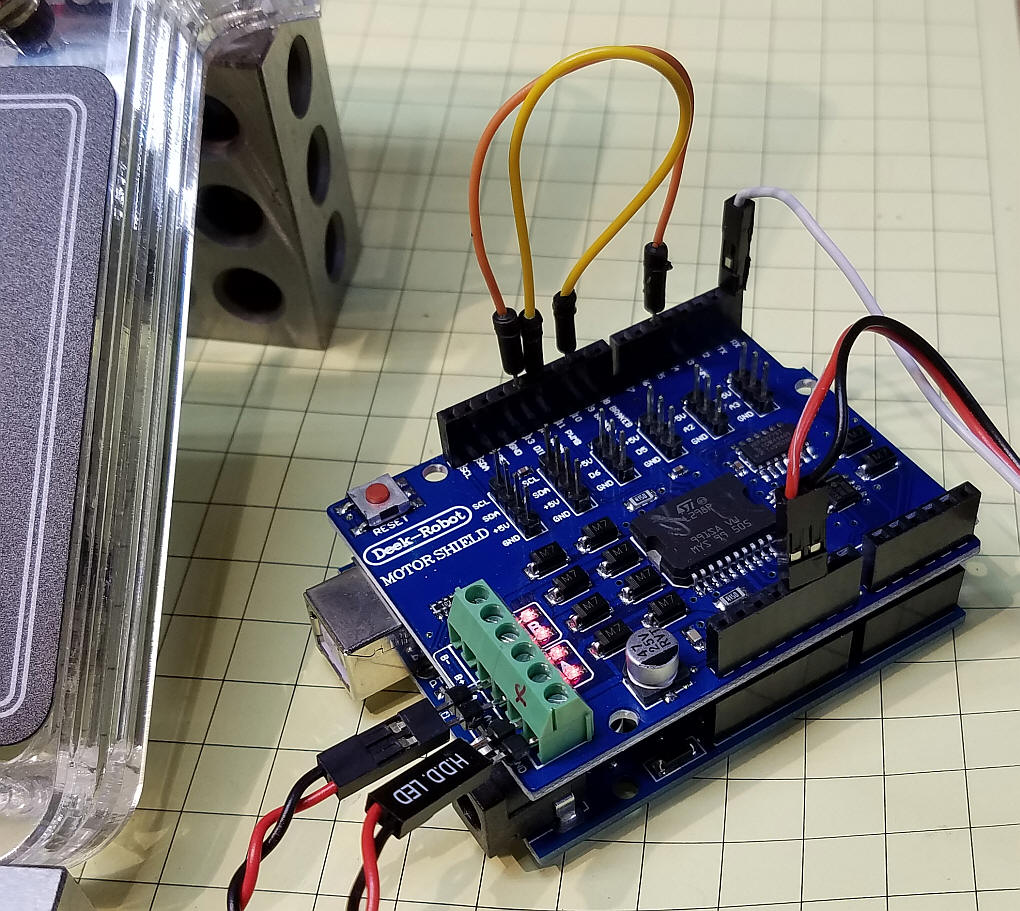Operation
To use the throttle connect +5 and ground from the Arduino Pro Mini to the +5 and ground pins on the DCC++ controller.  Connect the TX pin on the Pro Mini to the RX pin on the DCC++ controller.

Turn on the power and you will see an introductory screen followed by one that looks something like this: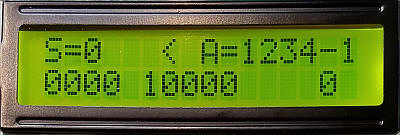You can press the right button (labeled select loco) to select one of the 4 loco addresses that can be stored.  In the photo above loco 1 has been chosen.

Press the left button (labeled loco/addr) and you can enter the 4 digit loco address.  Note that all 4 digits must be entered.  For example for address 3 press 0 then 0 then 0 then 3.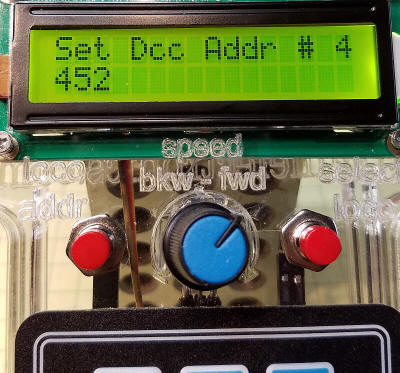Turn the potentiometer knob to the right (clockwise) to increase the speed forward and turn it left to increase the speed backwards.  The center position stops the locomotive.  This screen shows that the speed (S) is 43 and the direction (<) is backwards. The loco is #2 and its number is 2345Here loco #4, with ID 1451, is going forward (>) at a speed or 40.Pressing the "loco addr" button will shut the controller off stopping all trains.  As soon as you start up a train with the potentiometer the power will be restored.

Putting it Together
This view shows the LCD panel, two switches and potentiometer before they are wired to the Arduino Pro Mini.This interior view shows the wired prototype.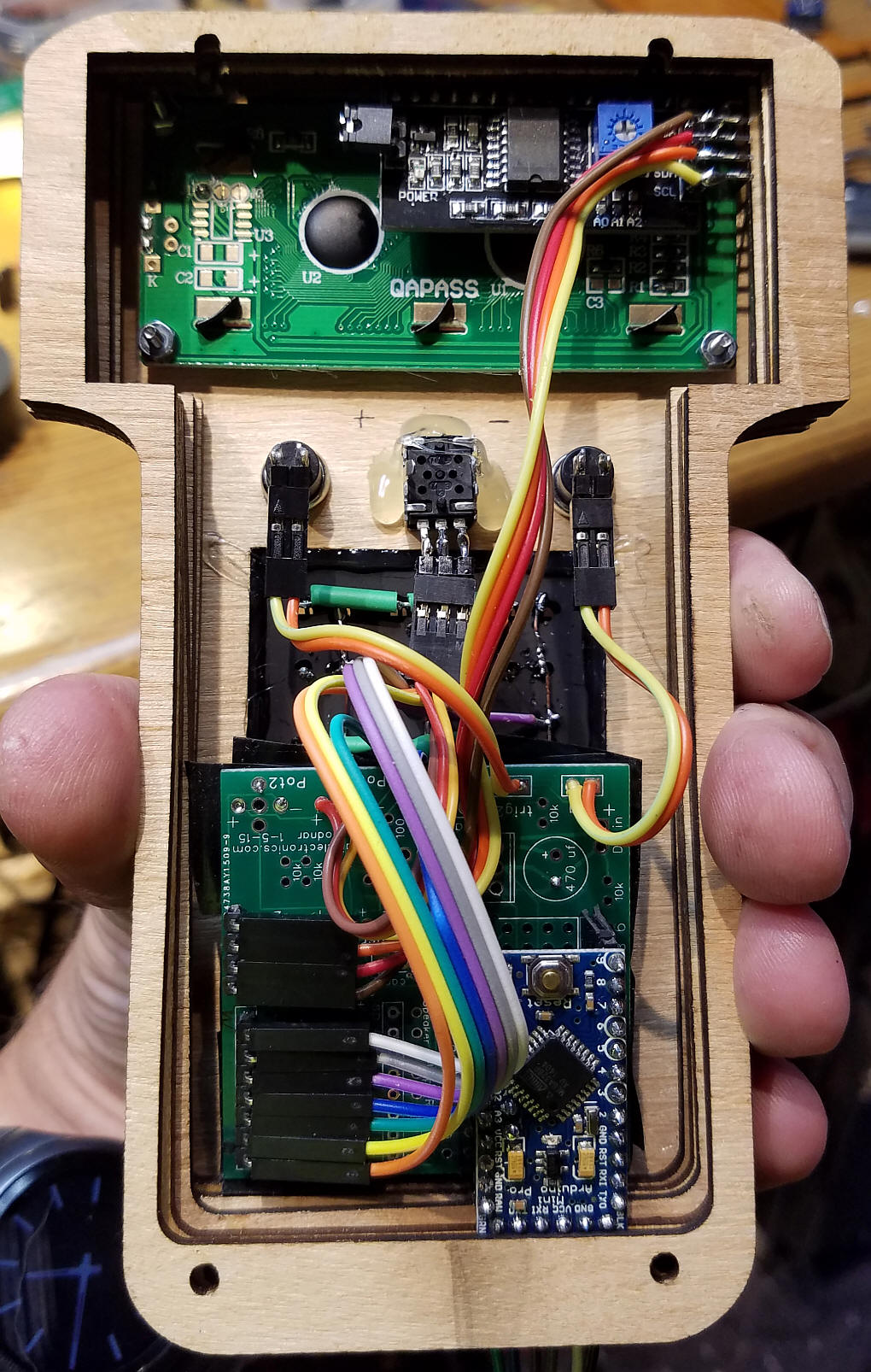The case for the two prototypes I have built were designed in CorelDraw and made with my laser cutter.  There are three different pieces.

 Front Panel (the green items are engraved, the others cut)Inside Spacers (thick enough for 5/8") (the notch at the bottom is only needed for one spacer)Back Panel (only one needed)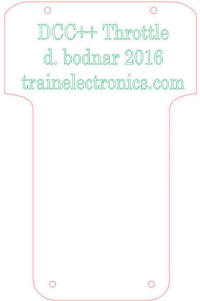Here are the six pieces that I used for the acrylic case (front, back, four spacers).  One spacer has a notch in it to allow the power/data cable to exit.This is a prototype that I made from 1/8" thick plywood.The keypad was made from a base plate of acrylic and 12 micro switches wired into a 3x4 matrix.

 Front view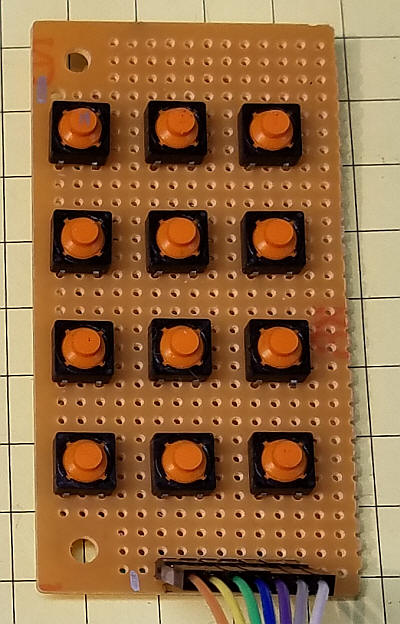Back view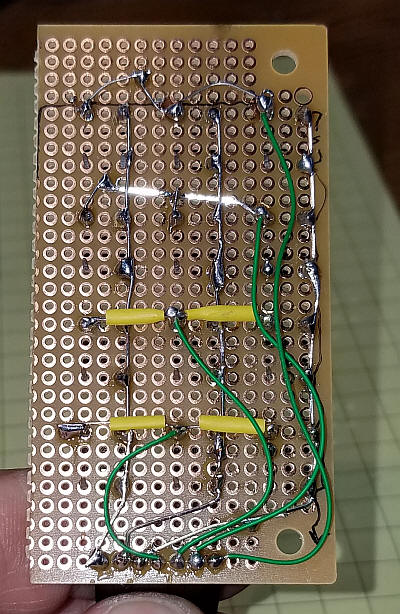This is a commercial unit that is functionally identical to the one I made. I got mine on eBayThis back view shows the matrix wiring of the commercial unit.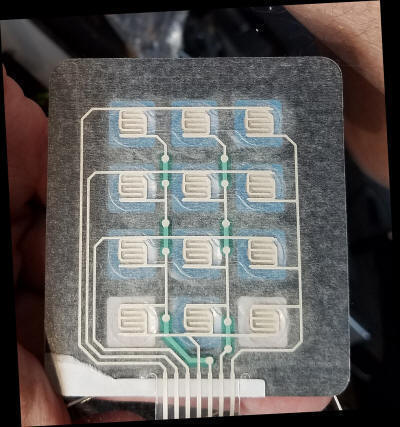This view shows the wiring of the 3x4 matrix on the test keypad.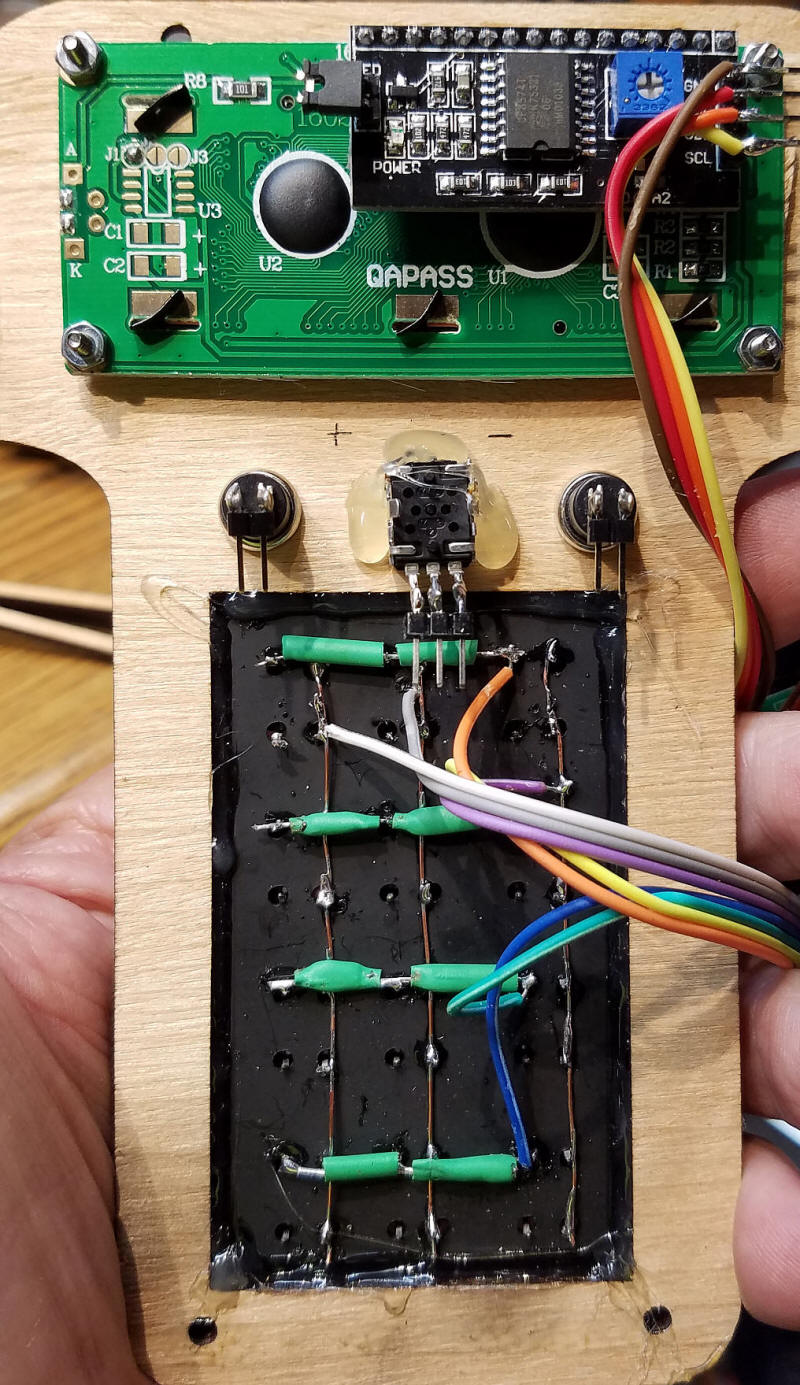The first prototype is shown here.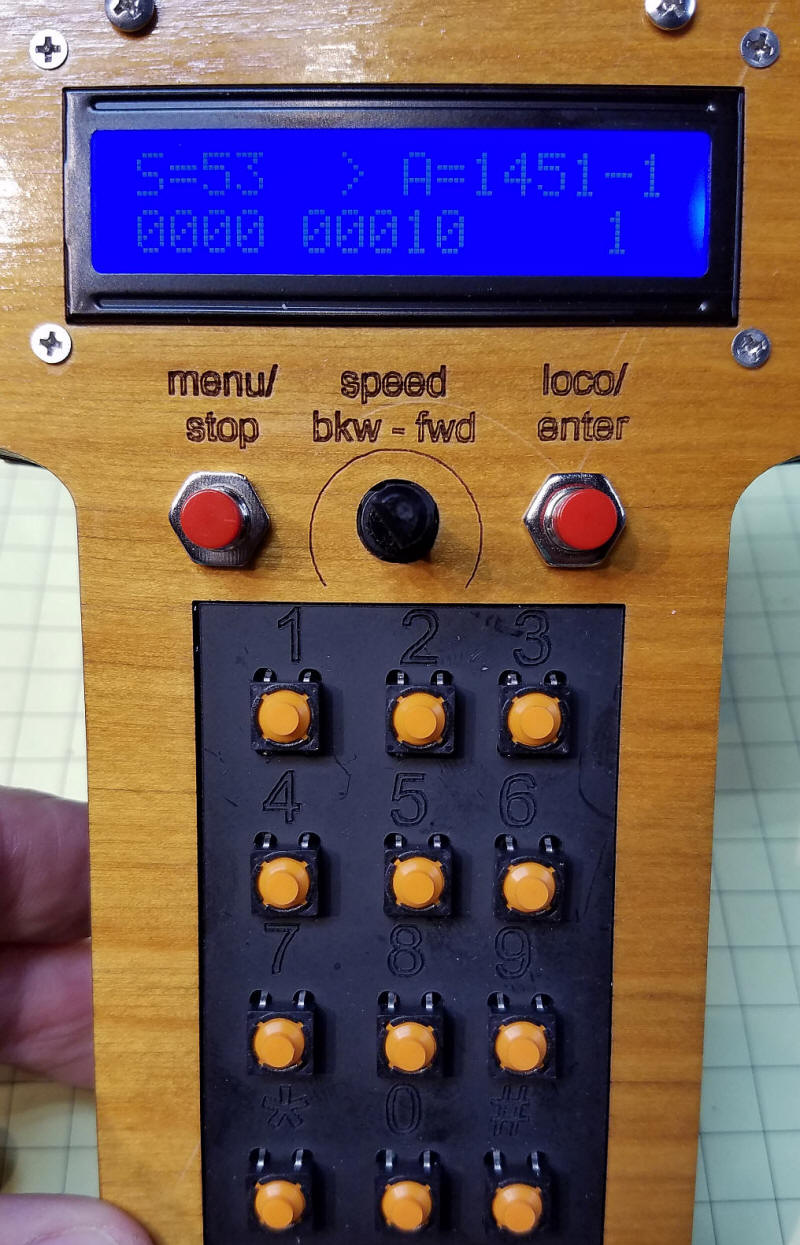The second prototype is shown here.  It uses a standard Arduino 3x4 matrix keypad.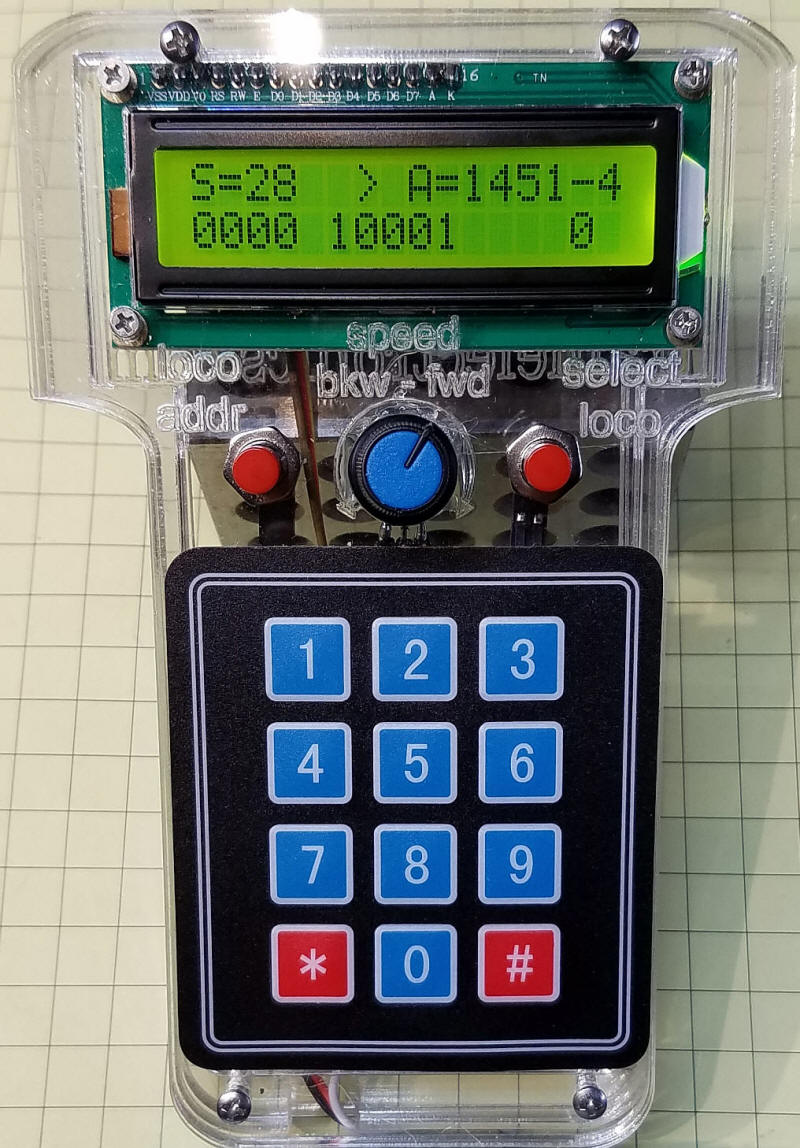Arduino Code
The latest version of the software is below.  Please note that it is currently under development and will change from time-to-time.  It is also likely to have bugs!

If you have difficulty compiling you can try my set of libraries which are available here:
http://trainelectronics.com/dcc_arduino/arduinoDCClibraries/libraries.zip

`DCC_Throttle-lcd-pot-keypad--v1-7F`
` Note:  Line    #include "Arduino.h"  Moved to top of code - some versions of the IDE may cause an error if it lower in the sketch. `
```
/*
VERSION WITH 3x4 KEYPAD, not capacitive touch unit
Modified to use * and # in place of two buttons

d. bodnar  revised 5-23-2016
To Do:
1.  LCD to show Loco #, speed, direction   L=5721 S=88 D=>    16 Characters
2.  LCD line two Function display    0000 0000 0000 ?????
3.  Left button calls up Menu
4.  Right button used to change loco in focus
5.  POT center = stop, CCW = reverse to max, CW = forward to max

*/
#include "Arduino.h"
byte Key;
#include<EEPROM.h>
char key ;
int LED = 13; // LED to blink when DCC packets are sent in loop
byte Fx = 0;
// Array set for 4 Loco2 - change the 7 numbers in the next 7 declarations
int maxLocos = 4;// number of loco addresses
int LocoAddress = {1830, 3, 999, 4444};
int LocoDirection = {1, 1, 1, 1};
int LocoSpeed = {0, 0, 0, 0};
byte LocoFN0to4;
byte LocoFN5to8;
byte Locofn9to12;// 9-12 not yet implemented
int xxxxx = 0;
int pot1Pin = A3;
int potValue = 0;
int NewPotValue = 0;
int potValueOld = 0;
int counter = 0;
int Trigger1 = 3;
int Trigger2 = 4;
int TrigVal1 = 0;
int TrigVal2 = 0;
int old_speed = 0;
int ZeroSpeedFlag = 0;
#include <Wire.h>
#include <LCD.h>
#include <LiquidCrystal_I2C.h>
#define BACKLIGHT_PIN     3
#define En_pin  2
#define Rw_pin  1
#define Rs_pin  0
#define D4_pin  4
#define D5_pin  5
#define D6_pin  6
#define D7_pin  7
LiquidCrystal_I2C	lcd(I2C_ADDR, En_pin, Rw_pin, Rs_pin, D4_pin, D5_pin, D6_pin, D7_pin);
int z = 0;
int powerTemp = 0;
int i = 0;
char VersionNum[] = "1.7F "; ///////////////////////// //////////////////////VERSION HERE///////
const byte ROWS = 4; //four rows
const byte COLS = 3; //three columns
char keys[ROWS][COLS] = {
{'1', '2', '3'},
{'4', '5', '6'},
{'7', '8', '9'},
{'*', '0', '#'}
};
byte rowPins[ROWS] = {5, 6, 7, 8 }; //{8,7,6,5 }; //connect to the row pinouts of the keypad
byte colPins[COLS] = {9, 10, 11}; // {11,10,9}; //connect to the column pinouts of the keypad
byte ledPin = 13;
boolean ledPin_state;
int debug = 0; // set to 1 to show debug info on serial port - may cause issues with DCC++ depending on what is sent

void setup() {
pinMode(Trigger1, INPUT);
pinMode(Trigger2, INPUT);
digitalWrite(Trigger1, HIGH);// turn on pullup resistors
digitalWrite(Trigger2, HIGH);// turn on pullup resistors
pinMode(LED, OUTPUT);
lcd.begin (16, 2); //  LCD is 16 characters x 2 lines
lcd.setBacklightPin(BACKLIGHT_PIN, POSITIVE);
lcd.setBacklight(HIGH);  // Switch on the backlight
lcd.home (); // go home
Serial.begin (115200);
lcd.setCursor(0, 0);
lcd.print("DCC++ Throttle");
lcd.setCursor(0, 1);
lcd.print("5-26-16 - v");
for (int i = 0; i < 4; i++) {
lcd.print(VersionNum[i]);
//delay(500);
}
Serial.print("5-22-2016  version ");
for (int i = 0; i < 4; i++) {
Serial.print(VersionNum[i]);
//delay(500);
}
if (debug == 1) Serial.println("");
Serial.print("<0>");// power off to DCC++ unit
delay(1500);

pinMode(ledPin, OUTPUT);              // Sets the digital pin as output.
digitalWrite(ledPin, HIGH);           // Turn the LED on.
ledPin_state = digitalRead(ledPin);   // Store initial LED state. HIGH when LED is on.

// showFirstLine();
lcd.clear();

}  // END SETUP

void loop() {
// if (key) {
if (key == 42) { // *
all2ZeroSpeeed();
key = 0;
}
// }
if (TrigVal1 == 0) {
all2ZeroSpeeed();
//   showFirstLine();
}
if (potValue != potValueOld) {
NewPotValue = 1;
//doMainLCD();
}
else NewPotValue = 0;
potValueOld = potValue;
potValue = (potValue / 4) - 128;
if (potValue <= 0) {
}
else LocoDirection[ActiveAddress] = 1; // forward
potValue = abs(potValue);
if (potValue >= 126) potValue = 126; // max is 127
if (potValue <= 3) potValue = 0; // set to zero if close to zero
if (NewPotValue == 1) {
doDCC();
doMainLCD();
NewPotValue = 0;
delay(50);
}
if (key == 35) { // #
//showFirstLine();
doMainLCD();
delay(200);
key = 0;
}
if (TrigVal2 == 0) {   // change loco # on right button press
//showFirstLine();
doMainLCD();
delay(200);
}
//    Serial.print("KKKKKKKKKKKKKKKKKEY =");
//    Serial.println(key);
if (key != 42 && key != 35 && key >= 40) {
//   if (key == 42) Serial.println("****************************");
//   Serial.print("KEY =");
//   Serial.println(key);
doFunction();
}
// doMainLCD();
}  //END LOOP

//START DO FUNCTION BUTTONS
int doFunction() {
key = key - 48 - 1; // convert from ascii value
lcd.setCursor (14, 1);       // go to end of 2nd line
///  lcd.print("FN code ");
lcd.print(key, DEC);
if (debug == 1) Serial.print("got a keypad button ");
if (debug == 1) Serial.println(key, DEC);
if (key <= 4) {
}
else {
}
}
doDCCfunction04();
if (debug == 1) Serial.println(" LocoFN0to4[ActiveAddress] d ");
if (debug == 1) Serial.println(" LocoFN0to4[ActiveAddress]");
}
if (key >= 5 && key <= 8) {
key = key - 5;
}
else {
}
}
doDCCfunction58();
if (debug == 1) Serial.println(" LocoFN5to8[ActiveAddress] d ");
if (debug == 1) Serial.println(" LocoFN5to8[ActiveAddress]");
}
if (key == -1)
{
lcd.setCursor (14, 1);       // go to end of 2nd line
///    lcd.print("FN code ");
lcd.print(key, DEC);
key = 0;
LocoFN0to4[ActiveAddress] = B00000000; //clear variables for which functions are set
doDCCfunction04();
doDCCfunction58();
delay(500);
key = 0;
}
key = 0;
// delay(500);
doMainLCD();
}
//END DO FUNCTION BUTTONS

void showFirstLine() {
// break;
if (debug == 1) Serial.println(" ");
lcd.setCursor(0, 0);
lcd.print("                ");// clear
lcd.setCursor(0, 0);
lcd.print("L");
lcd.print("=");

for (int zzz = 0; zzz <= 3; zzz++) {
if (debug == 1) Serial.print("add # ");
if (debug == 1) Serial.print(zzz + 1);
if (debug == 1) Serial.print(" ");

}

}

Serial.print("<0>");// power off to DCC++ unit
int total = 0;
counter = 0;
lcd.clear();
lcd.setCursor(0, 0);
if (debug == 1) Serial.println(" @ top");
do {
if (TrigVal2 == 0) break; // exit routine if right button pressed - ABORT new address
if (key) {
counter++;
int number =  key - 48;
total = total * 10 + number;
if (debug == 1) Serial.print("TOTAL= ");
if (debug == 1) Serial.println(total);
lcd.setCursor(0, 1);
if (total == 0) {   // print multiple zeros for leading zero number
for (int tempx = 1; tempx <= counter; tempx++) {
lcd.print("0");
}
}
else lcd.print(total);
if (debug == 1) Serial.print("Counter = ");
if (debug == 1) Serial.print(counter);
if (debug == 1) Serial.print("  key= ");
if (debug == 1) Serial.print(key);

if (debug == 1) Serial.print("   val =");
if (debug == 1) Serial.println(number);
}
}
while (counter <= 3); //  collect exactly 4 digits
//  while (  TrigVal2 == 1);
lcd.clear();
doMainLCD();
}

// Taking care of some special events.
case PRESSED:
if (key == '#') {
ledPin_state = digitalRead(ledPin);        // Remember LED state, lit or unlit.
}
break;

case RELEASED:
if (key == '*') {
digitalWrite(ledPin, ledPin_state);   // Restore LED state from before it started blinking.
}
break;

case HOLD:
if (key == '*') {
blink = true;    // Blink the LED when holding the * key.
}
break;
}
}

void doDCC() {
//Serial.print("d = ");
if (debug == 1) Serial.println(LocoDirection[ActiveAddress] );
Serial.print("<1>");
Serial.print("<t1 ");
Serial.print(" ");
Serial.print(" ");
Serial.write(">");
}

void doDCCfunction04() {
Serial.write("<f ");
Serial.print(" ");
int fx = LocoFN0to4[ActiveAddress] + 128;
Serial.print(fx);
Serial.print(" >");
}

void doDCCfunction58() {
Serial.write("<f ");
Serial.print(" ");
int fx = LocoFN5to8[ActiveAddress] + 176;
Serial.print(fx);
Serial.print(" >");
}

void all2ZeroSpeeed() {  // set flag to 1 to stop, set to 0 to restore
for (int tempx = 0; tempx <= maxLocos; tempx++) {
Serial.print("<t1 ");
Serial.print(" ");
if (ZeroSpeedFlag == 1) {
Serial.print(0);//LocoSpeed );
}
else Serial.print(LocoSpeed );
Serial.print(" ");
Serial.print(LocoDirection );
Serial.write(">");
}
}

int xxx = 0;
for (int xyz = 0; xyz <= maxLocos - 1; xyz++) {

if (debug == 1) Serial.println(" ");
if (debug == 1) Serial.print("loco = ");
if (debug == 1) Serial.print("  address# = ");
if (debug == 1) Serial.print(xyz + 1);
}
if (debug == 1) Serial.println(" ");
}

int xxx = 0;
for (int xyz = 0; xyz <= maxLocos - 1; xyz++) {
if (debug == 1) Serial.println(" ");
if (debug == 1) Serial.print("loco = ");
if (debug == 1) Serial.print("  address# = ");
if (debug == 1) Serial.print(xyz);
if (debug == 1) Serial.print(" msb ");
if (debug == 1) Serial.print(xxx);
if (debug == 1) Serial.print(" writing to ");
if (debug == 1) Serial.print(xyz * 2);
if (debug == 1) Serial.print(" and ");
if (debug == 1) Serial.print(xyz * 2 + 1);
EEPROM.write(xyz * 2, xxx);
xxx = LocoAddress[xyz] - (xxx * 256);
if (debug == 1) Serial.print(" lsb ");
if (debug == 1) Serial.print(xxx);
EEPROM.write(xyz * 2 + 1, xxx);
}
}

void doMainLCD() {
//lcd.setCursor(0, 0);
//lcd.print("               ");
lcd.setCursor(0, 0);
lcd.print("S=");
lcd.print("  ");
lcd.setCursor(6, 0);
if (LocoDirection[ActiveAddress] == 1 ) {
lcd.print(">");
}
else {
lcd.print("<");
}

lcd.setCursor(8, 0);

lcd.print("L=");
}
lcd.print("   ");
lcd.setCursor(14, 0);
lcd.print("#");
lcd.setCursor (15, 0);       // go to end of 1st line
lcd.setCursor(5, 1); // start of 2nd line
int tlen = temp.length() - 5;
lcd.print(temp.substring(tlen));
temp = "000" + String(LocoFN5to8[ActiveAddress], BIN);
tlen = temp.length() - 4;
lcd.setCursor(0, 1); // start of 2nd line
lcd.print(temp.substring(tlen));

}
```
```
```

Wireless Option

The throttle works very well with a 3 wire cable connection between it and the DCC++ unit.  You will recall that these three wires carry 5 volts DC, ground and the serial data that goes from the throttle TX pin to the DCC++ RX pin.

To make the unit wireless several changes have to be made.

• The case needs to be enlarged to accommodate a battery.  It was lengthened about 1"
• A battery must be added.  The longer case can accommodate many different batteries.  Any one that supplies from about 7-12 volts DC will work.  A standard 9 volt battery fits nicely and gives about 8 hours of continuous use.  Such batteries are generally not rechargeable and would have to be replaced often.  I opted to use two small cell phone batteries (3.7 volts each) wired in series to give a rechargeable 7.4 volt battery.
• A JST connector was added to the case.  It protrudes from the bottom of the case so that a charger can be plugged in.
• A power switch was added as well.  It is in the position that once was occupied by a pushbutton switch that was used to select a loco address.  Loco selection is not done with the # key on the keypad.  The DCC Address button (to the left of the potentiometer)  has also been moved to the * key.
• A pair of HC-12 modules were added.  One to the throttle and one to the DCC++ controller.
• The HC-12 modules both need to be configured for a baud rate of 115,200.  This procedure and other information about the HC-12 is on this web page:   http://trainelectronics.com/Arduino/HC-12-Serial_Radio/
• No software changes were made other than those needed to move the two buttons to the * and # keys on the keyboard.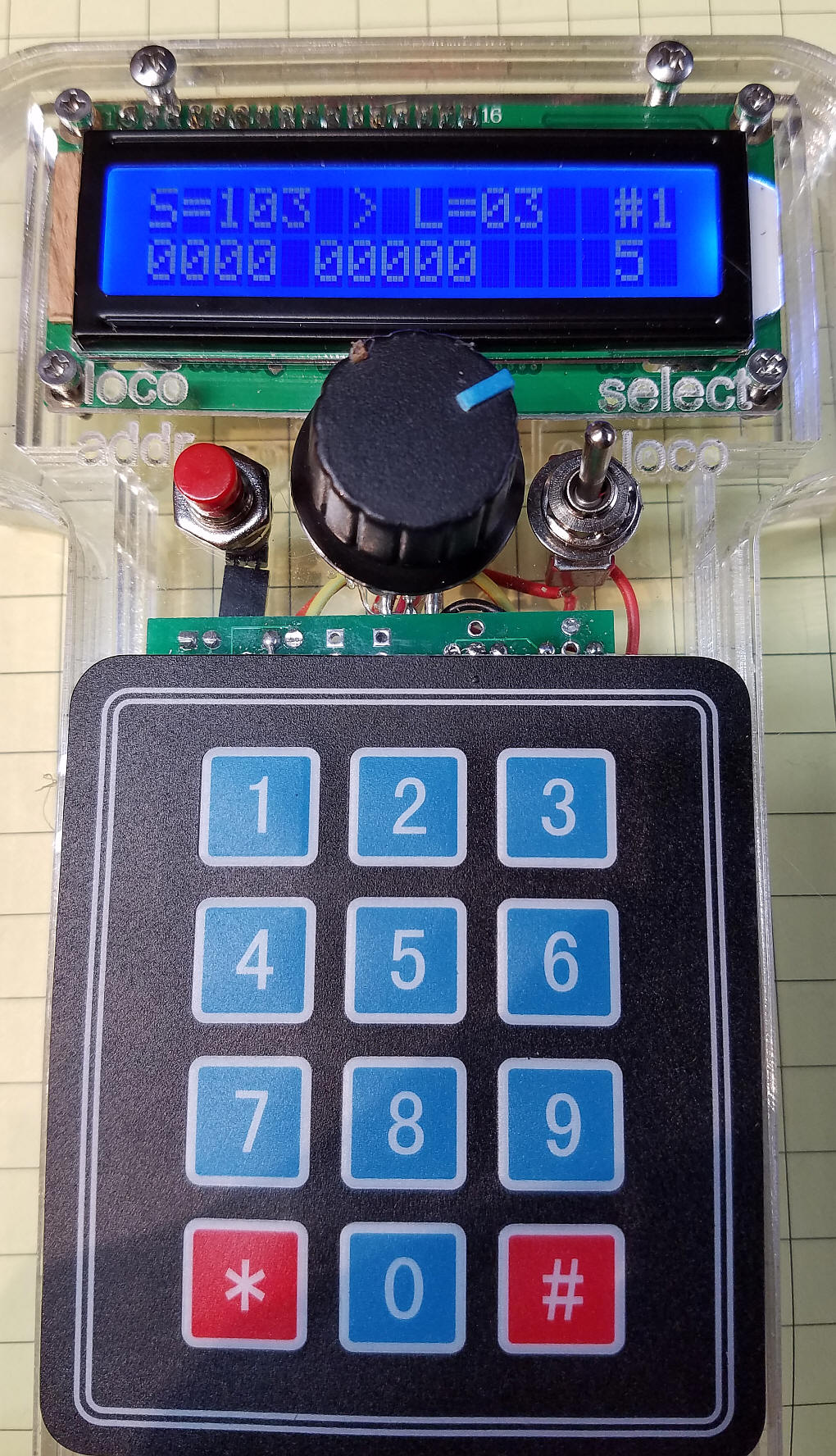This photo shows the battery at the bottom of the case.  You can also see the red JST connector that is used to charge the battery.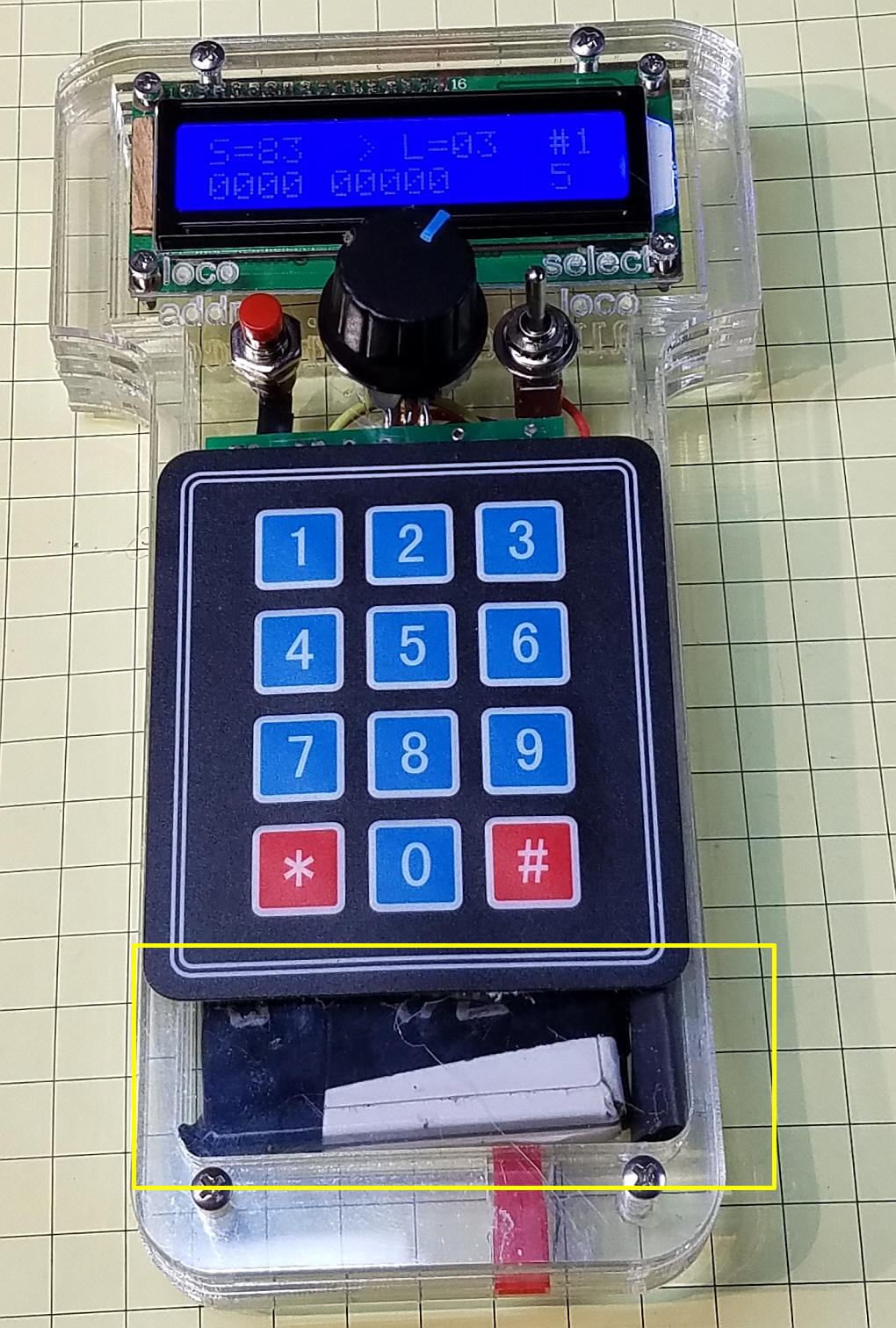This back view shows the full battery and the JST connector.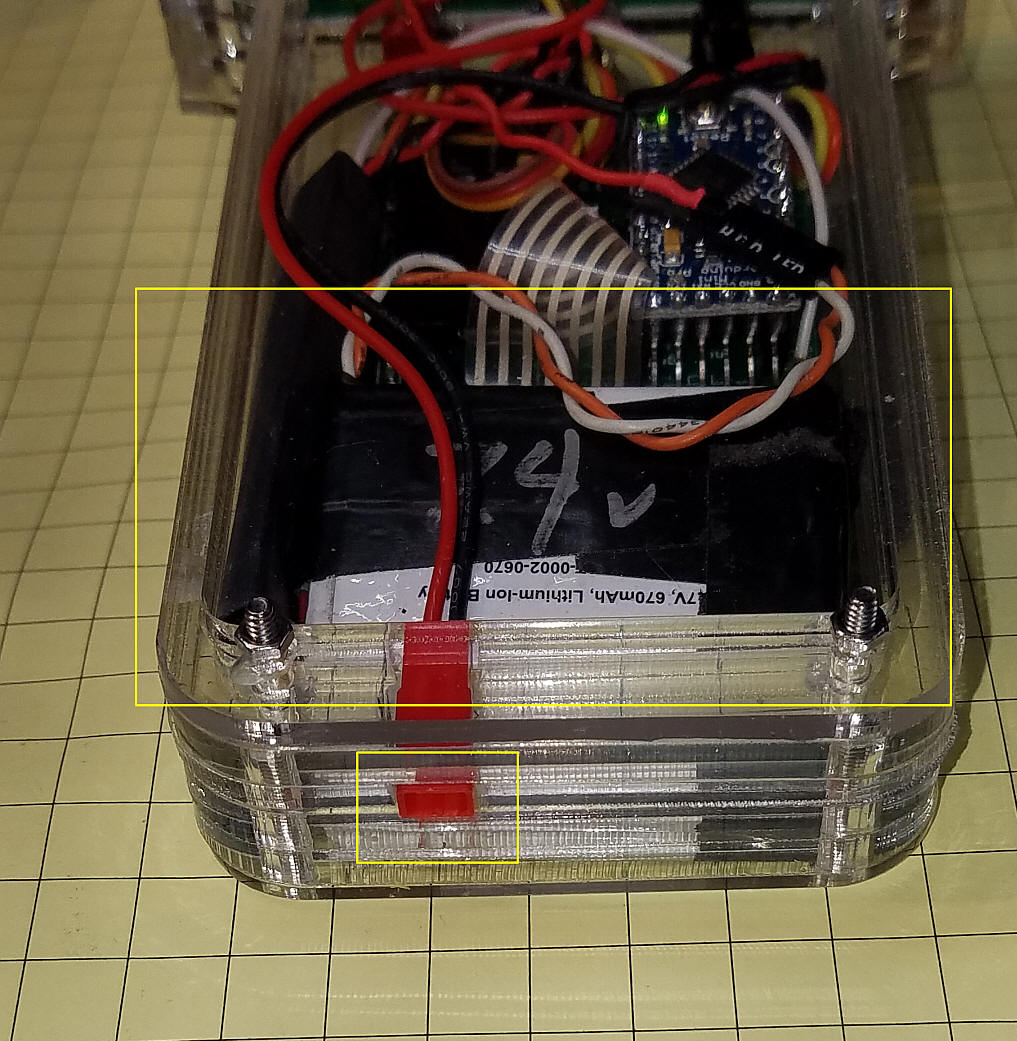The full back view clearly shows all of the  components.Wiring for Wireless
The batteries I am using have an internal charging circuit as do most cell phone batteries.  This allows the simplest of charging circuits.  I just send power (about 9 volts for a 7.2 volt battery) to the positive and negative terminals on the battery.  When the battery is charged it automatically stops charging.

A voltage regulator has been added to the circuit to drop the battery voltage to 5 volts for the throttle.  The only other change is connecting the HC-12 RX pin to the Arduino TX pin.

You need to add the other HC-12 to the DCC++ unit connecting it to +5 volts, ground and wiring its TX pin to the DCC++ Arduino's RX pin.

SIMPLE!Parts
The parts list is below.
• Arduino Pro Mini - eBay, Amazon
• 2 x 16 LCD display - eBay, Amazon
• 3x4 keypad - eBay, Amazon
• 7805 voltage regulator - various vendors
• 1uf tantalum capacitor (actually any value from 1 to abut 10 will work)
• 10k to 100k pot, linear taper - various vendors
• or... rotary encoder - eBay, Amazon
• HC-12 serial radio module - eBay, Amazon
• battery - 9 volt or two cell  phone batteries in series (7-10 volts total) - eBay, Amazon
• two switches (pushbutton) - various vendors

Rotary Encoder in place of Potentiometer
Some model railroaders prefer a rotary encoder over the potentiometer that is used in the above throttle.  This requires modification to the hardware and software.

The schematic shown below includes a rotary encoder that has a pushbutton switch built in.  The three pins shown in the photo are for the encoder.  There are two more pins on the other side for the button.Rotary Encoders like this one are available on eBay and Amazon.

You turn the knob on the encoder clockwise or counter clockwise to change speed up or down and push the knob in to change direction.  Note that the pushbutton part of the encoder includes a 10k pull-up resistor.  Make sure you use this rather than using the Arduino's capability of pulling a pin up internally.  That option does not work reliably while the 10k pull-up does work very well.

This schematic shows the wireless HC-12 units but the rotary encoder version of this throttle will work equally well when hard wired to the DCC++ controller.  The two 0.1 uf caps (C3 and C4) help to debounce the signal from the encoder.  They go between the outer pins and the center pin (ground).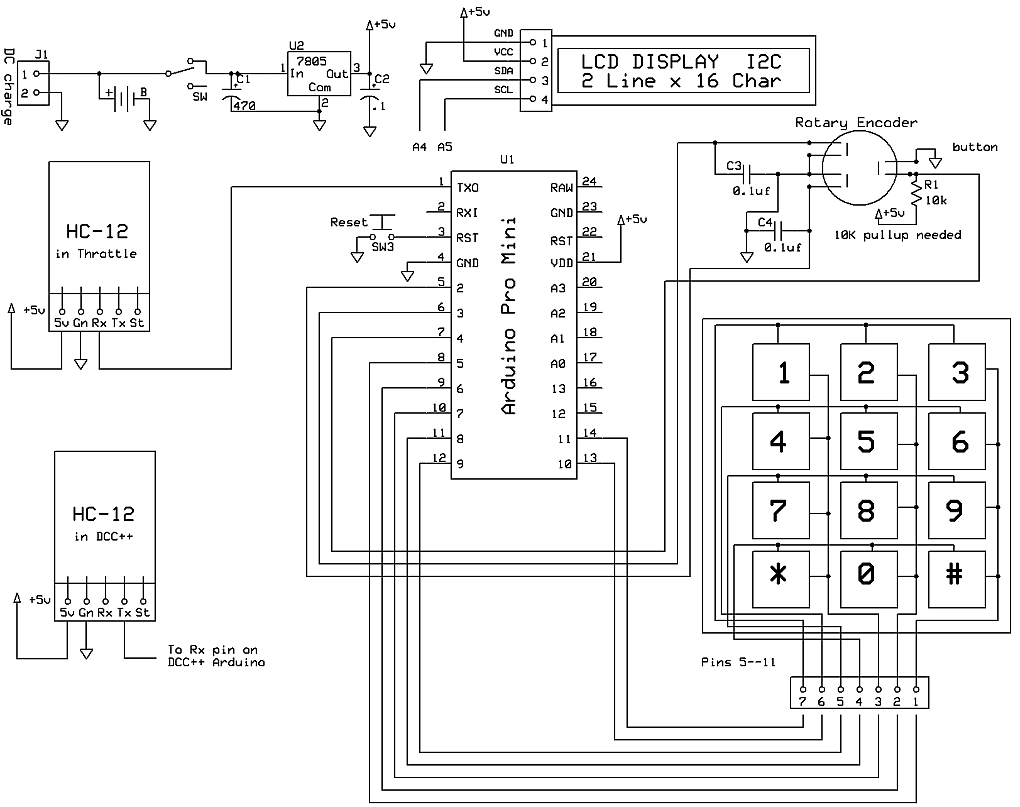An Unexpected Event
While using the wireless version of the throttle I discovered that the HC-12 transceiver  pairs appear to have an internal configuration that allows the receiver attached to the DCC++ unit to receive transmitted packets from two different throttles without any interference or parsing errors.  This behavior was quite unexpected but turns out to be a welcome thing!

This allows two throttles to control different trains on the same track at the same time.  I have not tried using 3 or more throttles but will once I build up additional throttles.

Software Changes in this Version
The throttle can handle up to 4 engines.  Pressing the # button changes the locomotive that is being controlled.  If you are using only 2 locomotives it can become tedious to press the # button several extra times to skip over the unused locomotive addresses.  To eliminate this problem you can change the number of locomotives that the throttle will control to 4, 3, 2 or 1.  To make this change press the button on the rotary encoder when turning on the throttle.  You will be shown the number of active addresses and will be asked to press 1, 2, 3 or 4 on the keypad to set the new number of locomotives.  The number of active addresses that you choose will be stored in the throttle and will be kept until you change it.

The maximum speed is limited to 126.  If you need to change this up or down you can change the value in this line - it is at about line 222 in the sketch.

`   encoderPos = constrain(encoderPos, 0, 126);`

Arduino Code for Rotary Encoder
The code is fairly complete but is sure to be updated after more testing.

If you have difficulty compiling you can try my set of libraries which are available here:
http://trainelectronics.com/dcc_arduino/arduinoDCClibraries/libraries.zip

DCC_Throttle-lcd-RotaryEncoder-keypad--v2-6a    -- version that allows you to set maximum number of locos (1-4)

Note:  Line    #include "Arduino.h"  Moved to top of code - some versions of the IDE may cause an error if it is lower in the sketch.

/*
d. bodnar  revised 6-16-2016
*/
#include "Arduino.h"
const int ledPin =  13;      // the number of the LED pin
int buttonPin = 4; // button on rotary
static int pinA = 2; // Rotary Encoder
static int pinB = 3; // Rotary Encoder
volatile byte aFlag = 0;
volatile byte bFlag = 0;
volatile byte encoderPos = 0;
volatile byte oldEncPos = 0;
int old_pos = encoderPos;
int dir = 0; // direction
int buttonState = 0;
int encoderChange = 0; // flag to show encoder changed
byte Key;
#include<EEPROM.h>
char key ;
int LED = 13; // LED to blink when DCC packets are sent in loop
byte Fx = 0;
// Array set for 4 Loco2 - change the 7 numbers in the next 7 declarations
int maxLocos = 4;// number of loco addresses
int LocoAddress = {1830, 3, 999, 4444};
int LocoDirection = {1, 1, 1, 1};
int LocoSpeed = {0, 0, 0, 0};
byte LocoFN0to4;
byte LocoFN5to8;
byte Locofn9to12;// 9-12 not yet implemented
int xxxxx = 0;
int pot1Pin = A3;
int potValue = 0;
int NewPotValue = 0;
int potValueOld = 0;
int counter = 0;
int Trigger1 = 3;
int Trigger2 = 4;
//int TrigVal1 = 0;
//int TrigVal2 = 0;
int old_speed = 0;
int ZeroSpeedFlag = 0;
#include <Wire.h>
#include <LCD.h>
#include <LiquidCrystal_I2C.h>
#define BACKLIGHT_PIN     3
#define En_pin  2
#define Rw_pin  1
#define Rs_pin  0
#define D4_pin  4
#define D5_pin  5
#define D6_pin  6
#define D7_pin  7
LiquidCrystal_I2C lcd(I2C_ADDR, En_pin, Rw_pin, Rs_pin, D4_pin, D5_pin, D6_pin, D7_pin);
int z = 0;
int powerTemp = 0;
int i = 0;
char VersionNum[] = "2.6a"; ///////////////////////// //////////////////////VERSION HERE///////
const byte ROWS = 4; //four rows
const byte COLS = 3; //three columns
char keys[ROWS][COLS] = {
{'1', '2', '3'},
{'4', '5', '6'},
{'7', '8', '9'},
{'*', '0', '#'}
};
byte rowPins[ROWS] = {5, 6, 7, 8 }; //{8,7,6,5 }; //connect to the row pinouts of the keypad
byte colPins[COLS] = {9, 10, 11}; // {11,10,9}; //connect to the column pinouts of the keypad
//byte ledPin = 13;
boolean ledPin_state;
unsigned long previousMillis = 0;        // will store last time LED was updated
unsigned long currentMillis = millis();
const long interval = 300;

int debug = 0; // set to 1 to show debug info on serial port - may cause issues with DCC++ depending on what is sent

void setup() {
pinMode(ledPin, OUTPUT);
pinMode(pinA, INPUT_PULLUP); // set pinA as an input, pulled HIGH to the logic voltage (5V or 3.3V for most cases)
pinMode(pinB, INPUT_PULLUP); // set pinB as an input, pulled HIGH to the logic voltage (5V or 3.3V for most cases)
pinMode(buttonPin, INPUT);
attachInterrupt(0, PinA, RISING); // set an interrupt on PinA, looking for a rising edge signal and executing the "PinA" Interrupt Service Routine (below)
attachInterrupt(1, PinB, RISING); // set an interrupt on PinB, looking for a rising edge signal and executing the "PinB" Interrupt Service Routine (below)
//  pinMode(Trigger1, INPUT);
//  pinMode(Trigger2, INPUT);
//  digitalWrite(Trigger1, HIGH);// turn on pullup resistors
//  digitalWrite(Trigger2, HIGH);// turn on pullup resistors
pinMode(LED, OUTPUT);
lcd.begin (16, 2); //  LCD is 16 characters x 2 lines
lcd.setBacklightPin(BACKLIGHT_PIN, POSITIVE);
lcd.setBacklight(HIGH);  // Switch on the backlight
lcd.home (); // go home
Serial.begin (115200);
lcd.setCursor(0, 0);
lcd.print("Hold button to");
lcd.setCursor(0, 1);
lcd.print("vary MaxLocos ");
lcd.print(maxLocos);
delay(1000);
// Serial.println(buttonState);
if (buttonState == LOW) {
getNumberOfLocos();
//    do {  // routine to stay here till button released & not toggle direction
//    }      while (buttonState == LOW);
}

lcd.clear();
lcd.print("DCC++ Throttle");
lcd.setCursor(0, 1);
lcd.print("6-11-16 v");
for (int i = 0; i < 4; i++) {
lcd.print(VersionNum[i]);
}

Serial.print("6-11-2016  version ");
for (int i = 0; i < 4; i++) {
Serial.print(VersionNum[i]);
}
if (debug == 1) Serial.println("");
Serial.print("<0>");// power off to DCC++ unit
delay(1500);
pinMode(ledPin, OUTPUT);              // Sets the digital pin as output.
digitalWrite(ledPin, HIGH);           // Turn the LED on.
ledPin_state = digitalRead(ledPin);   // Store initial LED state. HIGH when LED is on.
lcd.clear();
doMainLCD();
}  // END SETUP

void getNumberOfLocos() {
//maxLocos = 4;// number of loco addresses
lcd.clear();
lcd.setCursor(0, 0);
lcd.print("Loco # now = ");
lcd.print(maxLocos);
lcd.setCursor(0, 1);
lcd.print("new # (1-4) ");
do {
if (debug == 1) Serial.print("key = ");
if (debug == 1) Serial.println(key);
key = key - 48 - 1; // convert from ascii value
//Serial.println(key);
if (key == 1 || key == 2 || key == 3 || key == 4) { // 1-4
maxLocos = key + 1;
if (debug == 1)    Serial.print("new maxLocos = ");
if (debug == 1)  Serial.println(maxLocos);
lcd.print(maxLocos);
delay(1400);
break;
}
} while (key != 1 || key != 2 || key != 3 || key != 4);
}

void loop() {
/*  // thie routine refreshes DCC commands ever "interval" milliseconds
currentMillis = millis();
if (currentMillis - previousMillis >= interval) {
// save the last time
previousMillis = currentMillis;
doDCC();
}
*/
if (key == 42) { // *
all2ZeroSpeeed();
key = 0;
}
//NEW ROUTINE USING ROTARY ENCODER FOR SPEED AND BUTTON ON IT FOR DIRECTION
getEncoder();
if (encoderChange == 1) {
encoderChange = 0;
doDCC();
doMainLCD();
}
// Serial.println(buttonState);
if (buttonState == LOW) {
delay(50);
buttonState = digitalRead(buttonPin); // check a 2nd time to be sure
if (buttonState == LOW) {// check a 2nd time to be sure
digitalWrite(ledPin, LOW);
}
else digitalWrite(ledPin, HIGH);
doDCC();
doMainLCD();
do {  // routine to stay here till button released & not toggle direction
}      while (buttonState == LOW);
}
}
if (key == 35) { // #
doMainLCD();
delay(200);
key = 0;
doDCC();
}
if (key != 42 && key != 35 && key >= 40) {
doFunction();
}
}  //END LOOP

void getEncoder() {
if (oldEncPos != encoderPos) {
encoderPos = constrain(encoderPos, 0, 126);
oldEncPos = encoderPos;
encoderChange = 1; // flag to show a change took place
}
}//END GET ENCODER ROUTINE

//START DO FUNCTION BUTTONS
int doFunction() {
key = key - 48 - 1; // convert from ascii value
lcd.setCursor (14, 1);       // go to end of 2nd line
///  lcd.print("FN code ");
lcd.print(key, DEC + 1);
if (debug == 1) Serial.print("got a keypad button ");
if (debug == 1) Serial.println(key, DEC);
if (key <= 4) {
}
else {
}
}
doDCCfunction04();
if (debug == 1) Serial.println(" LocoFN0to4[ActiveAddress] d ");
if (debug == 1) Serial.print(LocoFN0to4[ActiveAddress], DEC);
if (debug == 1) Serial.println(" LocoFN0to4[ActiveAddress]");
}
if (key >= 5 && key <= 8) {
key = key - 5;
}
else {
}
}
doDCCfunction58();
if (debug == 1) Serial.print(LocoFN5to8[ActiveAddress], BIN);
if (debug == 1) Serial.println(" LocoFN5to8[ActiveAddress] d ");
if (debug == 1) Serial.print(LocoFN5to8[ActiveAddress], DEC);
if (debug == 1) Serial.println(" LocoFN5to8[ActiveAddress]");
}
if (key == -1)
{
lcd.setCursor (14, 1);       // go to end of 2nd line
///    lcd.print("FN code ");
lcd.print(key, DEC);
key = 0;
LocoFN0to4[ActiveAddress] = B00000000; //clear variables for which functions are set
doDCCfunction04();
doDCCfunction58();
delay(500);
key = 0;
}
key = 0;
// delay(500);
doMainLCD();
}//END DO FUNCTION BUTTONS

void showFirstLine() {
// break;
if (debug == 1) Serial.println(" ");
lcd.setCursor(0, 0);
lcd.print("                ");// clear
lcd.setCursor(0, 0);
lcd.print("L");
lcd.print(" = ");

for (int zzz = 0; zzz <= 3; zzz++) {
if (debug == 1) Serial.print("add # ");
if (debug == 1) Serial.print(zzz + 1);
if (debug == 1) Serial.print(" ");
}
}

Serial.print("<0>");// power off to DCC++ unit
int total = 0;
counter = 0;
lcd.clear();
lcd.setCursor(0, 0);
if (debug == 1) Serial.println(" @ top");
do {
//  if (TrigVal2 == 0) break;
if (key == '#' | key == '*') { //abort when either is hit
break;// exit routine if # button pressed - ABORT new address
}
if (key) {
counter++;
int number =  key - 48;
total = total * 10 + number;
if (debug == 1) Serial.print("TOTAL = ");
if (debug == 1) Serial.println(total);
lcd.setCursor(0, 1);
if (total == 0) {   // print multiple zeros for leading zero number
for (int tempx = 1; tempx <= counter; tempx++) {
lcd.print("0");
}
}
else lcd.print(total);
if (debug == 1) Serial.print("Counter = ");
if (debug == 1) Serial.print(counter);
if (debug == 1) Serial.print("  key = ");
if (debug == 1) Serial.print(key);
if (debug == 1) Serial.print("   val = ");
if (debug == 1) Serial.println(number);
}
}
while (counter <= 3); //  collect exactly 4 digits
lcd.clear();
doMainLCD();
}

// Taking care of some special events.
case PRESSED:
if (key == '#') {
ledPin_state = digitalRead(ledPin);        // Remember LED state, lit or unlit.
}
break;
case RELEASED:
if (key == '*') {
digitalWrite(ledPin, ledPin_state);   // Restore LED state from before it started blinking.
}
break;
case HOLD:
if (key == '*') {
blink = true;    // Blink the LED when holding the * key.
}
break;
}
}

void doDCC() {
if (debug == 1) Serial.println(LocoDirection[ActiveAddress] );
Serial.print("<1>");
Serial.print("<t1 ");
Serial.print(" ");
Serial.print(" ");
Serial.println(">");
}

void doDCCfunction04() {
Serial.write("<f ");
Serial.print(" ");
int fx = LocoFN0to4[ActiveAddress] + 128;
Serial.print(fx);
Serial.print(" >");
}

void doDCCfunction58() {
Serial.write("<f ");
Serial.print(" ");
int fx = LocoFN5to8[ActiveAddress] + 176;
Serial.print(fx);
Serial.print(" >");
}

void all2ZeroSpeeed() {  // set flag to 1 to stop, set to 0 to restore
for (int tempx = 0; tempx <= maxLocos; tempx++) {
Serial.print("<t1 ");
Serial.print(" ");
if (ZeroSpeedFlag == 1) {
Serial.print(0);//LocoSpeed );
}
else Serial.print(LocoSpeed );
Serial.print(" ");
Serial.print(LocoDirection );
Serial.write(">");
}
}

int xxx = 0;
for (int xyz = 0; xyz <= maxLocos - 1; xyz++) {
if (debug == 1) Serial.println(" ");
if (debug == 1) Serial.print("loco = ");
if (debug == 1) Serial.print("  address# = ");
if (debug == 1) Serial.print(xyz + 1);
}
if (debug == 1) Serial.println(" ");
if (debug == 1) Serial.print("EEPROM maxLocos = ");
if (debug == 1) Serial.println(maxLocos);
if (maxLocos >= 4) maxLocos = 4;
}

int xxx = 0;
for (int xyz = 0; xyz <= maxLocos - 1; xyz++) {
if (debug == 1) Serial.println(" ");
if (debug == 1) Serial.print("loco = ");
if (debug == 1) Serial.print("  address# = ");
if (debug == 1) Serial.print(xyz);
if (debug == 1) Serial.print(" msb ");
if (debug == 1) Serial.print(xxx);
if (debug == 1) Serial.print(" writing to ");
if (debug == 1) Serial.print(xyz * 2);
if (debug == 1) Serial.print(" and ");
if (debug == 1) Serial.print(xyz * 2 + 1);
EEPROM.write(xyz * 2, xxx);
xxx = LocoAddress[xyz] - (xxx * 256);
if (debug == 1) Serial.print(" lsb ");
if (debug == 1) Serial.print(xxx);
EEPROM.write(xyz * 2 + 1, xxx);
}
EEPROM.write(20, maxLocos);
}

void doMainLCD() {
lcd.setCursor(0, 0);
lcd.print("S=");
lcd.print(" ");
lcd.setCursor(5 , 0);
if (LocoDirection[ActiveAddress] == 1 ) {
lcd.print(" > ");
}
else {
lcd.print(" < ");
}
lcd.setCursor(8, 0);
lcd.print("L=");
}
lcd.print("   ");
lcd.setCursor(14, 0);
lcd.print("#");
lcd.setCursor (15, 0);       // go to end of 1st line
lcd.setCursor(5, 1); // start of 2nd line
int tlen = temp.length() - 5;
lcd.print(temp.substring(tlen));
temp = "000" + String(LocoFN5to8[ActiveAddress], BIN);
tlen = temp.length() - 4;
lcd.setCursor(0, 1); // start of 2nd line
lcd.print(temp.substring(tlen));
}

void PinA() {
cli(); //stop interrupts happening before we read pin values
reading = PIND & 0xC; // read all eight pin values then strip away all but pinA and pinB's values
if (reading == B00001100 && aFlag) { //check that we have both pins at detent (HIGH) and that we are expecting detent on this pin's rising edge
if (encoderPos != 0)encoderPos --; //decrement the encoder's position count
bFlag = 0; //reset flags for the next turn
aFlag = 0; //reset flags for the next turn
}
else if (reading == B00000100) bFlag = 1; //signal that we're expecting pinB to signal the transition to detent from free rotation
sei(); //restart interrupts
}

void PinB() {
cli(); //stop interrupts happening before we read pin values
reading = PIND & 0xC; //read all eight pin values then strip away all but pinA and pinB's values
if (reading == B00001100 && bFlag) { //check that we have both pins at detent (HIGH) and that we are expecting detent on this pin's rising edge
encoderPos ++; //increment the encoder's position count
bFlag = 0; //reset flags for the next turn
aFlag = 0; //reset flags for the next turn
}
else if (reading == B00001000) aFlag = 1; //signal that we're expecting pinA to signal the transition to detent from free rotation
sei(); //restart interrupts
}

` `
This is the CorelDraw file that is used to make the case.  The front (bottom right), back (top center) and cutout for the power plug (top left) are made from 1/8" Plexiglas and the other three sections are made from 1/4" Plex.  You can download the file here:  DCC_Arduino/DCC++/Throttle/Throttle-encoder-wireless.cdr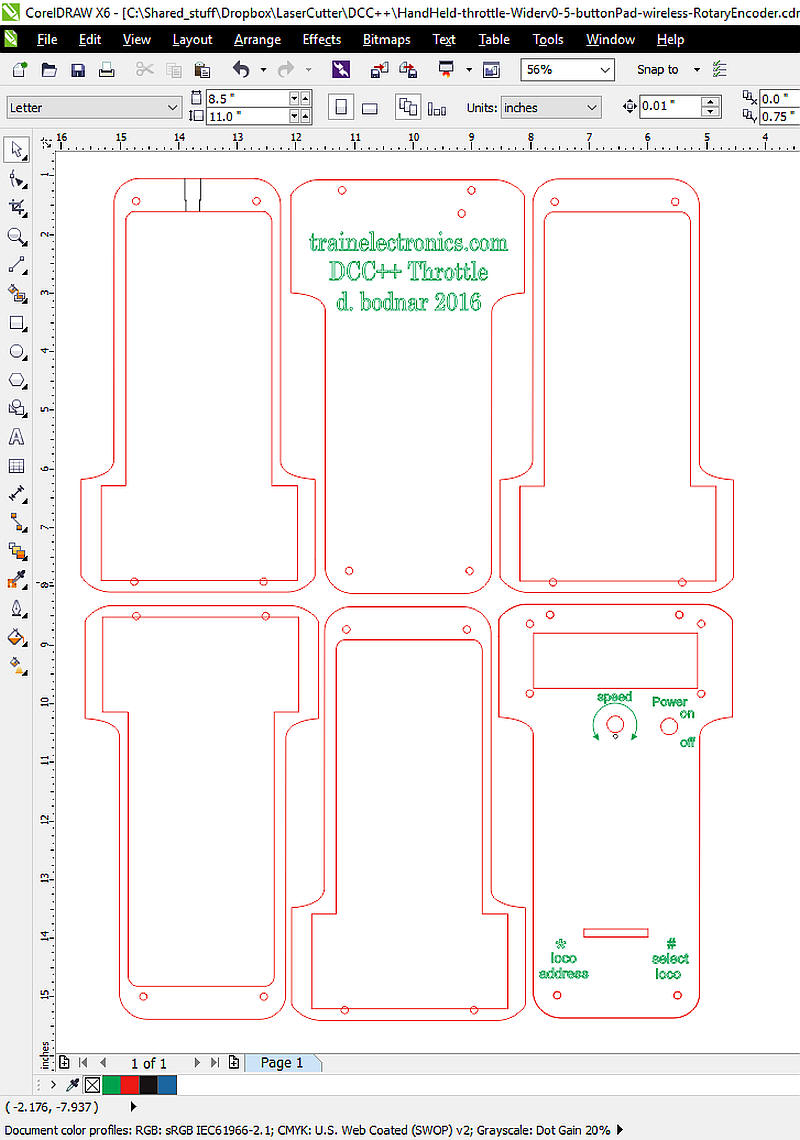Building from a Modified Circuit Board
The throttles shown above were built on a circuit board that was designed for an MP3 player that was controlled by the Arduino Pro Mini.  With some minor change it fits the throttle nicely.

The LCD connects to pins A4 and A5 which have to be accessed by a 2 pin header as is shown circled in yellow.  A matching pair of pins are soldered to the bottom of the Arduino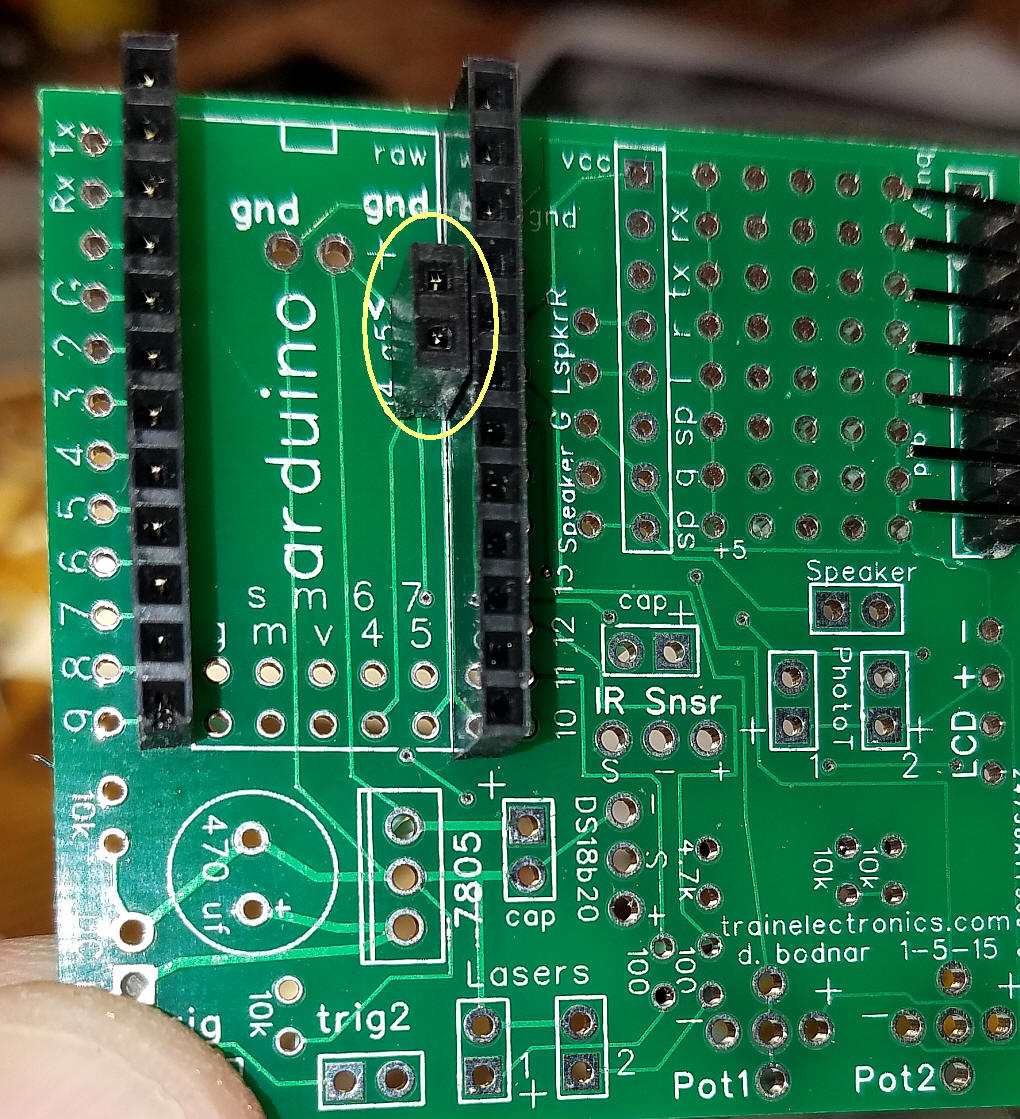The wiring for the keypad is shown here.  The schematic shows the pin numbers.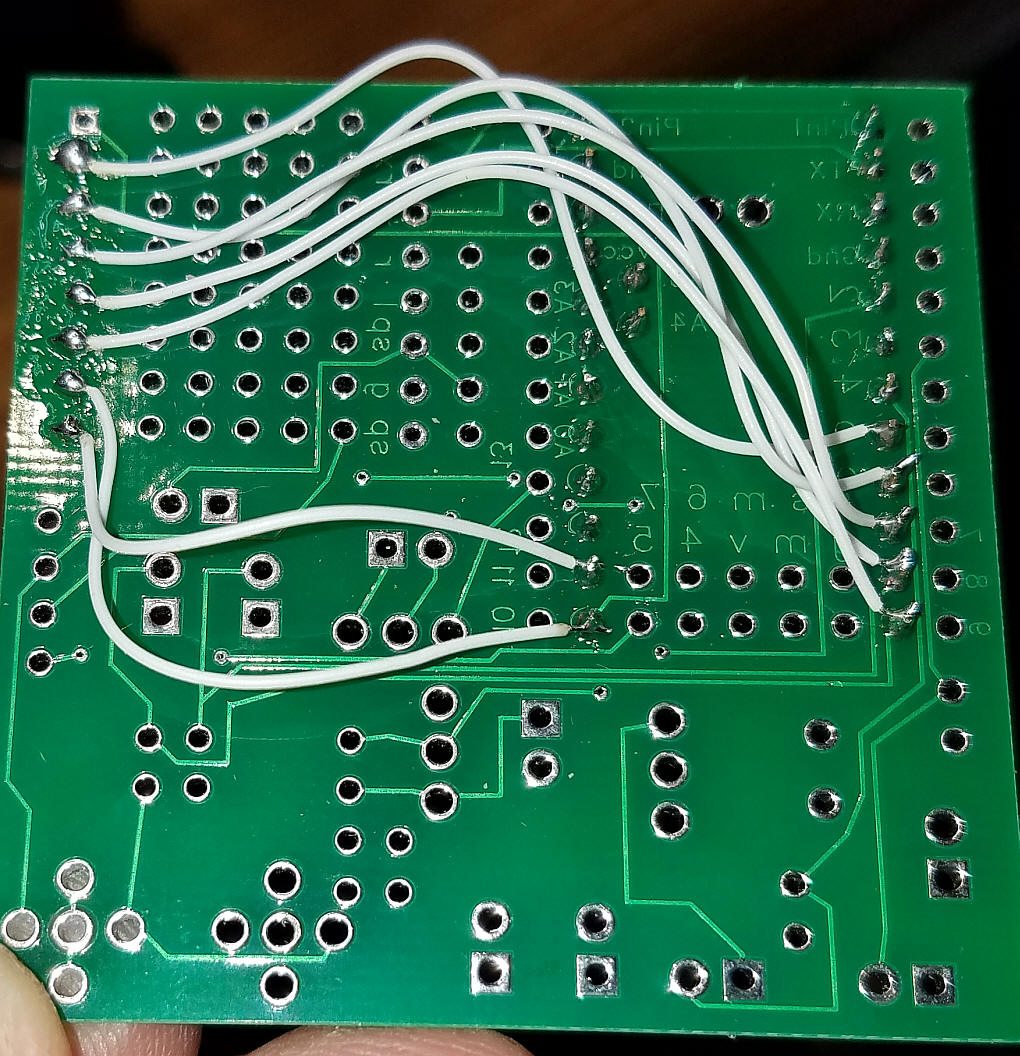The encoder and its button connect as shown here.  The four wires to the left go to the LCD.The wiring for the encoder includes two 0.1 uF caps each wired to an encoder data pin and ground.  If you prefer these can be added to the circuit board.  One pin of the button (the top two pins) goes to the board (white wire) while the other goes to ground (center pin on the 3 pin end of the encoder)A 10K resistor goes on the board as shown.  It pulls the button on the encoder high.  Note the cut trace at top, center.